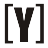# HTML Color Codes

#FF0000 are example of HTML color code of RED color, #00FF00 are for GREEN color and #0000FF are for BLUE color. HTML color code format contains of symbol “#” and 6 digit (numbers / letters), this format are hexadecimal numeral system, for example: “FF” in hex format represent 255 in decimal.

How does HTML color work? each two hex digits (#”XX” “XX” “XX“) are represent colours Red Green Blue (R=0-255, G=0-255, B=0-255).

How to convert HTML color codes to RGB? First take a look at the chart below.

 Hexadecimal 0 1 2 3 4 5 6 7 8 9 A B C D E F Decimal 0 1 2 3 4 5 6 7 8 9 10 11 12 13 14 15
Example:
HEX: #FF6F61 convert to RGB?
First you need to convert hex digit into decimal.
F=15, F=15, 6=6, F=15, 6=6 and 1=1

Each 2 digits convert to R / G / B value.
Hex: #FF6F61
FF = 15 and 15 in decimal
6F = 6 and 15 in decimal
61 = 6 and 1 in decimal

Conversion:
R value (FF): (15 x 16) + 15 = 255 (R)
G value (6F): (6 x 16) + 15 = 111 (G)
B value (61): (6 x 16) + 1 = 97 (B)

#FF6F61 = R: 255, G: 111, B: 97

Color Hex Code
#FF6F61
Decimal Code
(R,G,B)
#FF6F61 rgb(255,111,97)

## HTML Color Codes Picker

Insert (Hex) HTML color code:

## HTML Color Charts

### Color Code:YoosFuhl.com Would you like to receive notifications on latest updates? No Yes